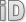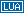# PlotType (Civ5 Type)

This page is a part of the Lua and UI Reference (Civ5).The `PlotType` pseudo-type is actually a regular integer. Pseudo-types do not exist in Lua, they only serve a documentation purpose on the wiki!Integers labeled as `PlotType` corresponds to the constants defined in the PlotTypes Lua enumeration.

# Lua: the PlotTypes enumeration

Firaxis provides a Lua enumeration named `PlotTypes`. This is just a regular global table. Its keys are the constants' names and the pairs the corresponding values.

Below are the values found in this enumeration. ``` ```

``` Key Value "NO_PLOT" -1 "PLOT_MOUNTAIN" 0 "PLOT_HILLS" 1 "PLOT_LAND" 2 "PLOT_OCEAN" 3 "NUM_PLOT_TYPES" 4 ```

# Examples

Here is how to use the LUA enumeration to retrieve the ID from the type. Those examples will return and assign the integer value 0.

```local id = PlotTypes.PLOT_MOUNTAIN
local id = PlotTypes["PLOT_MOUNTAIN"]```

# Used by

 `SetPlotTypes(table(int => PlotType) plotTypes)` `PlotType` `Plot:GetPlotType()` `Plot:SetPlotType(PlotType terrain, bool recalculateAreas = nil, bool rebuildGraphics) = nil)`

The initial version of this page was created by the Civ5 API Bot, see the Civ5 API Reference FAQ. Some of the texts come from the 2kgames' wiki and most of code samples are copyrighted to Firaxis.
Functions' signatures were either copied from the 2kgames' wiki, or infered from the Lua source files and the binaries. Errors are possible.
Contributors may find help in the Contributors guide to the Civ5 API.